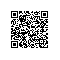# C# Lambda表达式详解，及Lambda表达式树的创建

Lambda表达式

"Lambda表达式"是一个匿名函数，是一种高效的类似于函数式编程的表达式，Lambda简化了开发中需要编写的代码量。它可以包含表达式和语句，并且可用于创建委托或表达式目录树类型，支持带有可绑定到委托或表达式树的输入参数的内联表达式。所有Lambda表达式都使用Lambda运算符=>，该运算符读作"goes to"。Lambda运算符的左边是输入参数(如果有)，右边是表达式或语句块。Lambda表达式x => x * x读作"x goes to x times x"。可以将此表达式分配给委托类型，如下所示：

delegate int del(int i);
static void Main(string[] args)
{

del myDelegate = x => x * x;
int j = myDelegate(5); //j = 25

}

using System.Linq.Expressions;

namespace ConsoleApplication1
{

class Program
{
static void Main(string[] args)
{
Expression<del> myET = x => x * x;
}
}

}

1、表达式Lambda

表达式位于 => 运算符右侧的 lambda 表达式称为“表达式 lambda”。 表达式 lambda 会返回表达式的结果，并采用以下基本形式：

   (input parameters) => expression

(x, y) => x == y

(int x, string s) => s.Length > x

() => SomeMethod()

2、语句Lambda

(input parameters) => {statement;}

delegate void TestDelegate(string s);

TestDelegate myDel = n => { string s = n + " " + "World"; Console.WriteLine(s); };
myDel("Hello");

        List<string> Citys= new List<string>()
{
"BeiJing",
"ShangHai",
"Tianjin",
"GuangDong"
};
var result = Citys.First(c => c.Length > 7);

public void LambdaFun(string str,Func<string,string> func)
{
Console.WriteLine(func(str));
}

LambdaFun("BeiJing 2013", s =>

     {
if (s.Contains("2013"))
{
s = s.Replace("2013", "2014");
}
return s;
});

lambda表达式树动态创建方法

 static void Main(string[] args)
{
//i*j+w*x
ParameterExpression a = Expression.Parameter(typeof(int),"i");   //创建一个表达式树中的参数，作为一个节点，这里是最下层的节点
ParameterExpression b = Expression.Parameter(typeof(int),"j");
BinaryExpression r1 = Expression.Multiply(a,b);    //这里i*j,生成表达式树中的一个节点，比上面节点高一级

ParameterExpression c = Expression.Parameter(typeof(int), "w");
ParameterExpression d = Expression.Parameter(typeof(int), "x");
BinaryExpression r2 = Expression.Multiply(c, d);

Expression<Func<int, int, int, int, int>> lambda = Expression.Lambda<Func<int, int, int, int, int>>(result,a,b,c,d);

Console.WriteLine(lambda + "");   //输出‘(i,j,w,x)=>((i*j)+(w*x))’，z对应参数b，p对应参数a

Func<int, int, int, int, int> f= lambda.Compile();  //将表达式树描述的lambda表达式，编译为可执行代码，并生成该lambda表达式的委托；

Console.WriteLine(f(1, 1, 1, 1) + "");  //输出结果2
}使用钉钉扫一扫加入圈子
+ 订阅